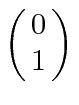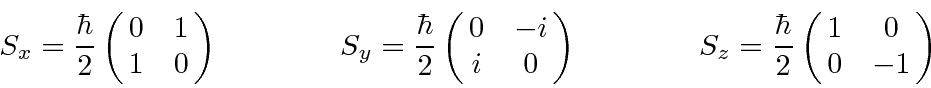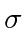## Spin 1/2 and other 2 State Systems

The angular momentum algebra defined by the commutation relations between the operators requires that the total angular momentum quantum number must either be an integer or a half integer. The half integer possibility was not useful for orbital angular momentum because there was no corresponding (single valued) spherical harmonic function to represent the amplitude for a particle to be at some position.

The half integer possibility is used to represent the internal angular momentum of some particles. The simplest and most important case is spin one-half. There are just two possible states with different z components of spin: spin up, with z component of angular momentum, and spin down, with. The corresponding spin operators areThese satisfy the usual commutation relations from which we derived the properties of angular momentum operators.

It is common to define the Pauli Matrices,, which have the following properties.The last two lines state that the Pauli matrices anti-commute. Thematrices are the Hermitian, Traceless matrices of dimension 2. Any 2 by 2 matrix can be written as a linear combination of thematrices and the identity.

Jim Branson 2013-04-22